• python画分段函数图像
千次阅读
2022-04-11 15:43:49
import matplotlib.pyplot as plt
import numpy as np
x = np.linspace(-10, 10, 100)
y = [i if (i>0) else 0 for i in x]
plt.figure()
plt.plot(x, y)
plt.title("relu")
plt.xlabel("np")
plt.ylabel("relu")
plt.show()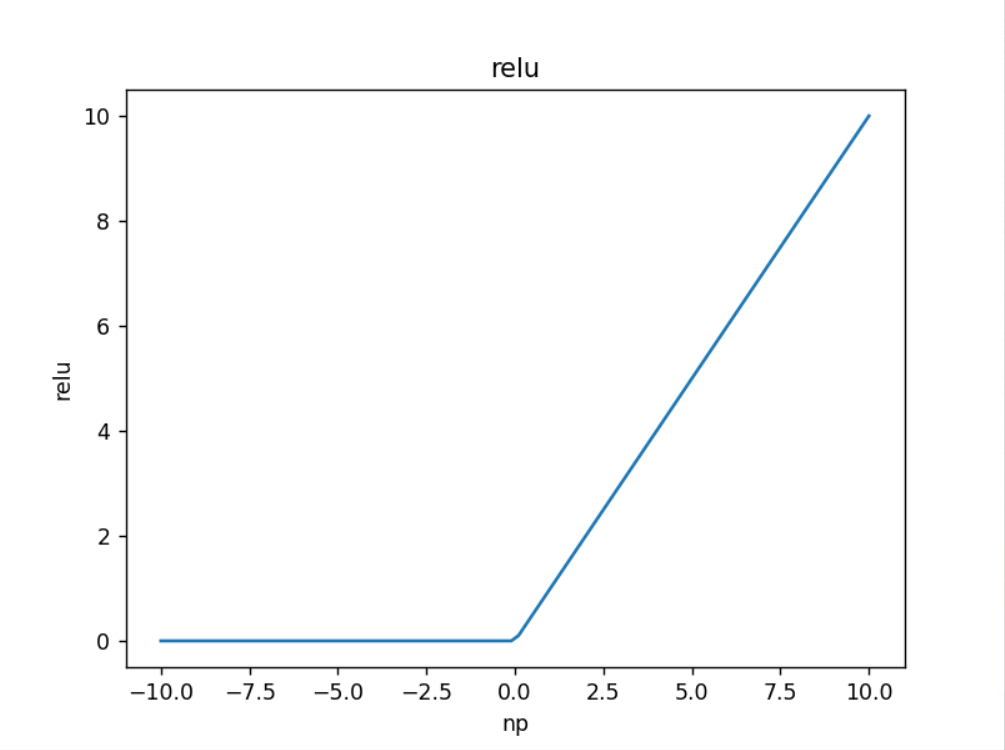更多相关内容
• python分段画图

目录

需求

参考示例

总结

# 需求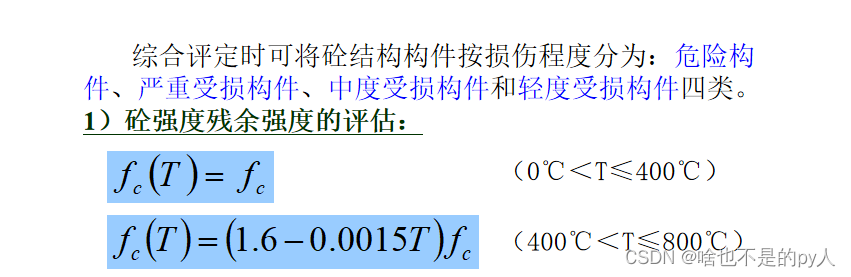画一个分段函数

先新建文件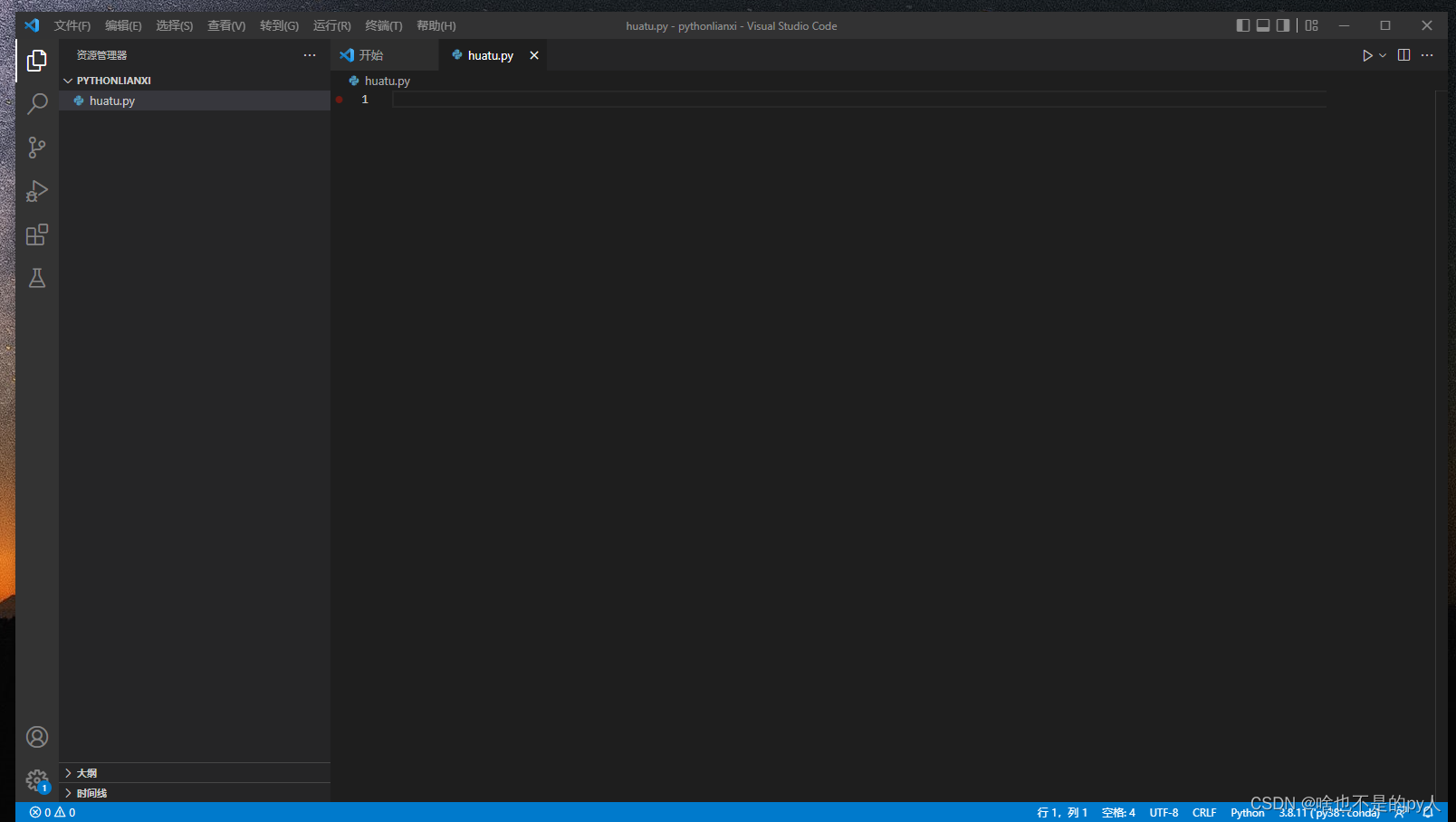先跑了一个一元一次函数实例

from mpl_toolkits.mplot3d import Axes3D
import numpy as np
from matplotlib import pyplot as plt
plt.rcParams['font.sans-serif'] = ['SimHei'] #用来正常显示中文标签
plt.rcParams['axes.unicode_minus'] = False #用来正常显示负号
# 一元一次函数图像
x = np.arange(-10, 10, 0.1)
y = 2 * x
plt.xlabel('x')
plt.ylabel('y')
plt.title("残余强度变化")
plt.plot(x, y)
plt.show()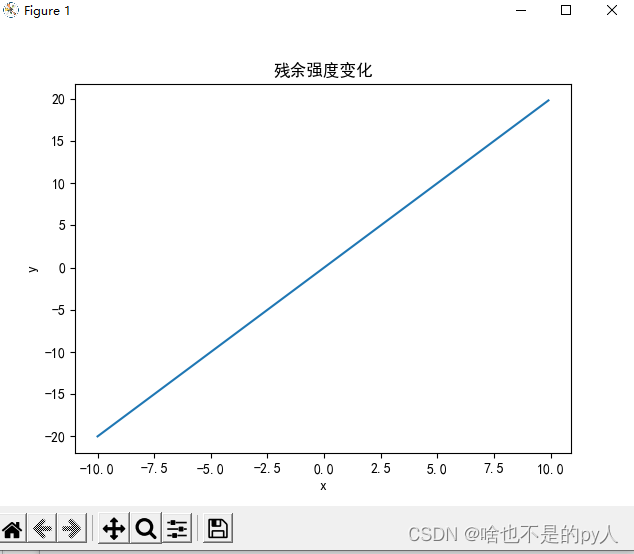然后开始设计分段函数

# 参考示例

直接def一个函数

算出y的所有数

然后把砼残余强度绘制出来

from mpl_toolkits.mplot3d import Axes3D
import numpy as np
from matplotlib import pyplot as plt
plt.rcParams['font.sans-serif'] = ['SimHei'] #用来正常显示中文标签
plt.rcParams['axes.unicode_minus'] = False #用来正常显示负号

def triangle_wave(x, c):
if x < c:
return 1
elif x >= c:
return (400*(1.6-0.0015*x))/400

x = np.linspace(0, 600, 1000)
y = np.array([triangle_wave(t, 400) for t in x])

plt.xlabel('温度')
plt.ylabel('残余抗压强度比值')
plt.title("残余强度变化")
plt.plot(x, y)
plt.show()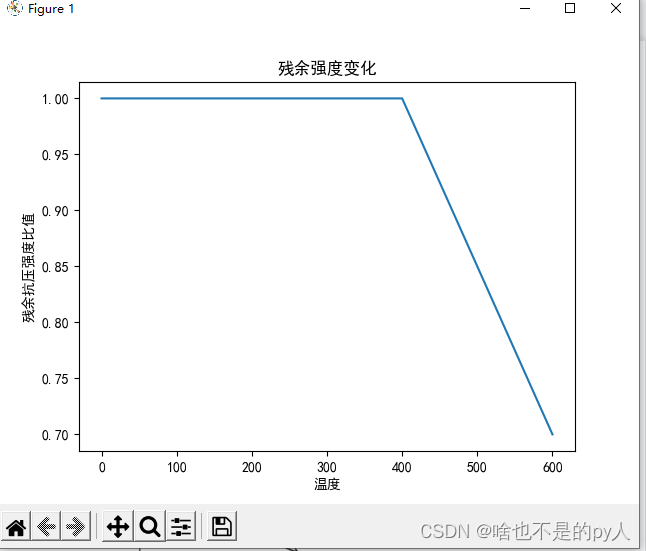还有钢筋残余强度变化

from mpl_toolkits.mplot3d import Axes3D
import numpy as np
from matplotlib import pyplot as plt
plt.rcParams['font.sans-serif'] = ['SimHei'] #用来正常显示中文标签
plt.rcParams['axes.unicode_minus'] = False #用来正常显示负号
def triangle_wave(x, c):
if x < c:
return 3.35
elif x >= c:
return 3.35*(1.32-0.00164*x)
x = np.linspace(0, 700, 1000)
y = np.array([triangle_wave(t, 200) for t in x])
plt.xlabel('温度')
plt.ylabel('残余抗压强度比值')
plt.title("钢筋残余强度变化-xx作业")
plt.plot(x, y)
plt.show()

绘制出图像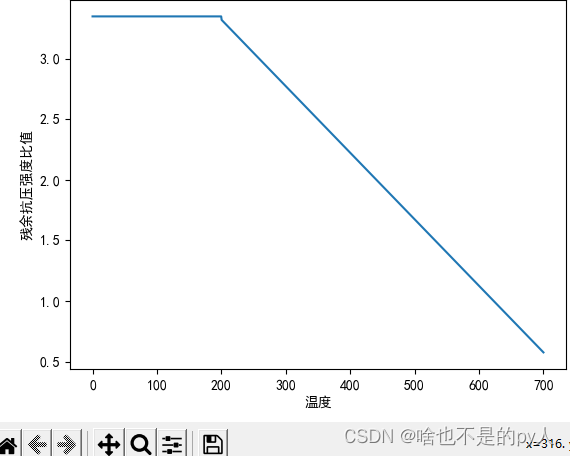# 总结

其实plt功能包的功能就是对应x，y的点绘制出函数

所以在此之前只要输出对应y值和x值

然后用x、y绘制出来即可

展开全文开发语言
• ## 通过Python绘制分段函数

万次阅读 多人点赞 2020-06-17 12:47:16
如何利用python绘制分段函数 下面通过整理的两个例子对两种方法进行说明： 例子1 主要思路是将函数y在各区间的值表示出来。 首先导包 from matplotlib import pyplot as plt import numpy as np 其次，确定定义域...

# 如何利用python绘制分段函数

下面通过整理的两个例子对两种方法进行说明：

## 例子1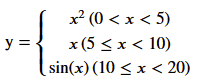主要思路是将函数y在各区间的值表示出来。
首先导包

from matplotlib import pyplot as plt
import numpy as np


其次，确定定义域为0~20，总共插值有1000个点

x = np.linspace(0,20,1000)


然后，为了表示分段函数y,通过列表来设置各段的函数自变量x的值

interval0 = [1 if (i<5) else 0 for i in x]
interval1 = [1 if (i>=5 and i<10) else 0 for i in x]
interval2 = [1 if (i>=10) else 0 for i in x]

y = x**2* interval0 + x * interval1 + np.sin(x)*interval2

plt.plot(x,y)
plt.show()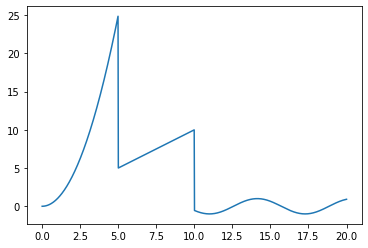## 例子2

如果需要画一个周期三角波形，如下图所示：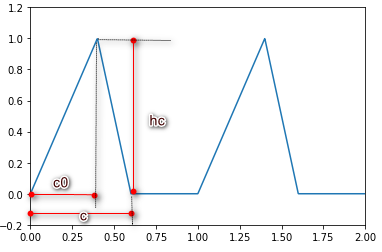该怎么做呢？基本想法是将该曲线用一个函数表示出来，函数如下

def triangle_wave(x, c, c0, hc):
x = x - int(x)  #三角波周期为1 因此只取小数部分进行计算
if x < c0:
return x / c0 * hc
elif x >= c:
return 0.0
else:
return (c-x)/(c-c0)*hc


注：因为图像是一个周期为1的函数，所以在计算函数值时，利用x在1以内的值即可，所以取小数。

from matplotlib import pyplot as plt
import numpy as np

def triangle_wave(x, c, c0, hc):
x = x - int(x)  #三角波周期为1 因此只取小数部分进行计算
if x < c0:
return x / c0 * hc
elif x >= c:
return 0.0
else:
return (c-x)/(c-c0)*hcx

x = np.linspace(0, 2, 1000)
y = np.array([triangle_wave(t, 0.6, 0.4, 1.0) for t in x]) #根据x计算各点函数值

plt.figure()
plt.plot(x, y)
plt.ylim(-0.2, 1.2)   #限制y的范围
plt.xlim(0, 2)        #限制x的范围
plt.show()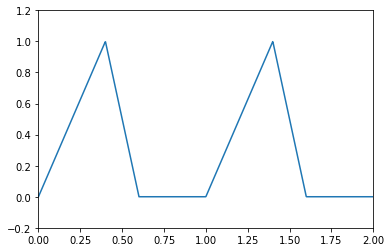## 总结

• 方法1实际上是将要绘制的函数分解为三个区间函数的叠加。三个区间函数在各自有定义的范围内计算函数值，在无定义的地方直接令函数值为0，从而达到计算分段函数的目的。
• 方法2实际是直接计算分段函数的各点函数值，个人感觉是通用的。
• 方法2其实可以实现例子1。过程类似，只是修改triangle_wave(x, c, c0, hc)函数为函数function()如下所示，
from matplotlib import pyplot as plt
import numpy as np

def function(x, x1, x2): # x1与x2是分段点位置
if x < x1:
return  x**2
elif x >= x2:
return np.sin(x)
else:
return x

x = np.linspace(0, 20, 1000)
y = np.array([function(t, 5, 10) for t in x]) #根据x计算各点函数值

plt.figure()
plt.plot(x, y)
plt.ylim(-3, 30)   #限制y的范围
plt.xlim(0, 22)   #限制x的范围
plt.show()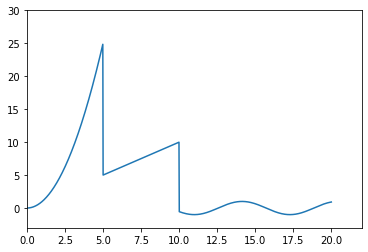更多精彩，请👇，还有更多小技巧和您交流。展开全文• 绘制分段函数：   首先导入matplotlib和numpy包： import matplotlib.plot as plt import numpy as np 规定x范围0-100,1000个点： x = np.linspace(0,100,1000) 设置区间： interval0 = [1 if (i&lt;...

绘制分段函数：

首先导入matplotlib和numpy包：

import matplotlib.plot as plt
import numpy as np

规定x范围0-100,1000个点：

x = np.linspace(0,100,1000)

设置区间：

interval0 = [1 if (i<30) else 0 for i in x]
interval1 = [1 if (i>=30 and i<50) else 0 for i in x]
interval2 = [1 if (i>=50) else 0 for i in x]

列函数式：

y = np.cos(x)* interval0 + x * interval1 + np.sin(x)*interval2

绘图显示：

plt.plot(x,y)
plt.show()

结果：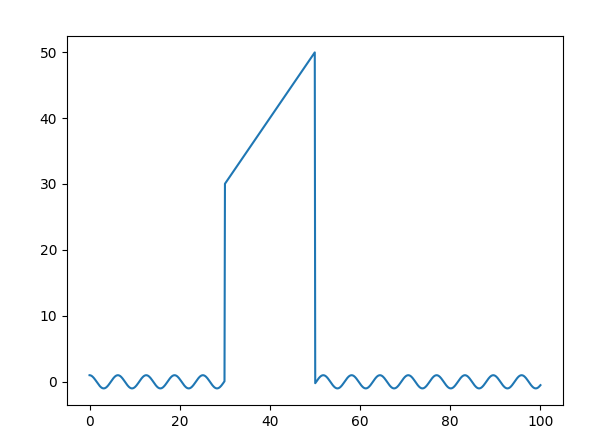展开全文matplotlib
• 分段函数图像画如下函数图像f ( x ) = { − 3 x + 1 ( x ≤ − 1 2 ) 3 + x ( − 1 2 ) 3 x − 1 ( x ≥ 2 ) f(x)=\left\{代码实现import matplotlib.pyplot as pltimport numpy as npt = np.arange(-3, 4, 0.01)y_...
• 写在前面高三数学中有几类比较特殊的分段函数，它们的图像画法很特殊，特做以收集整理。其中需要注意的是，分段函数的分支$$f(x)=f(x+k)$$和$$f(x)=f(x)+k$$和$$f(x)=af(x+k)$$的图像的做法。周期性+左右平移例1...
• python分段函数怎样编写？python编写分段函数的要领：1.绘制分段函数：y=4sin(4πt)-sgn(t-0.3)-sgn(0.72-t)代码：#!/usr/bin/python# -*- coding:utf-8 -*-import numpy as npimport matplotlib.pyplot as plt#绘制...
• 一、函数说明在使用python...本次针对数值实验中两个较为复杂的函数，使用其构建分段函数图像。二、图像代码2.11、函数公式：y=4sin(4πt)-sgn(t-0.3)-sgn(0.72-t)2.12、代码如下：import numpy as npimport matplo...
• from matplotlib import ...def matplotlibshow(a, b, c, d, e): # 定义新的函数 x = np.linspace(-25, 0, 1000) # 确定图像中定义域的取值范围 interval0 = [1 if (i <= a) else 0 for i in x] interval1 = .开发语言
• 本文实例为大家分享了python使用Matplotlib绘制分段函数的具体代码，供大家参考，具体内容如下环境Python3Mac OS代码# coding:utf-8"""Author: roguesirDate: 2017/8/30GitHub: ...
• 本文实例为大家分享了Python实现分段线性插值的具体代码，供大家参考，具体内容如下 函数： 算法 这个算法不算难。甚至可以说是非常简陋。但是在代码实现上却比之前的稍微麻烦点。主要体现在分段上。 图像效果 ...
• python下绘制分段函数，主要涉及到逻辑处理的问题，例如绘制下面的分段函数：⎧⎩⎨⎪⎪sin(x),x≤2cos(x),2
• 我试图用Numpy来拟合分段函数和绝对值。在数学函数是x<；p：y=1+p*abs（（size+x-p）/size-size/2）x>；=p：y=1+p*绝对值（（x-p）/尺寸-尺寸/2）下面是我的Python函数：fitfunc = lambda p, ...
• # 圆心坐标 x = np.arange(a - r, a + r, 0.01) y = b + np.sqrt(r ** 2 - (x - a) ** 2) axes.plot(y, x) # 右半部 k=k+20 def main():#总函数 global axes fig = plt.figure() axes = fig.add_subplot(111) lin() ...
•Matplotlib
• 方便起见，在这里使用一个分段函数的例子：计算三角波形（例子取自张若愚的《Python科学计算》） 三角波形具有周期性，因此我们只需要考虑0~1之间的这个范围就可以了，在这个范围里，又分了三个不同的表达式。 ...Numpy select where
• C语言编程题 有一分段函数如下，要求用scanf函数输入整数x的值，求y值并在屏幕上输出。 有分段函数如下，编程实现输入整www.zhiqu.org 时间： 2020-11-22#include int main(){ int x; double y; scanf("%d",&x)...
• （1）插值一般要求插值函数经过所有的样本点，形成复杂的插值函数。插值有两大基本思路：思路1：已知n个样本点，基于样本数据计算出唯一的插值函数f(x)，使得f(x)经过每个样本点，再根据f(x)计算新的x-new对应的y值...
• ## python绘制指数函数

千次阅读 2020-12-06 11:20:12
上一期，我们介绍了指数函数的形和质以及运算法则，其中有两个非常漂亮的指数函数图就是用python的matplotlib出来的。这一期，我们将要介绍如何利用python绘制出如下指数函数。图 1 a>1我们知道当0 ，指数函数 ...
• 为了绘制C空间投影(theta1,theta2,L1)，我们可以使用python中的matplotlib进行三维曲面的绘制。其中，L1=cos(θ1)+cos(θ2)L1=cos(\theta_1)+cos(\theta_2)L1=cos(θ1​)+cos(θ2​) from matplotlib import pyplot ...开发语言
• 如图，x轴是时间，y,z轴表示变量，随着时间是上升——平稳——下降的趋势，怎么求出y和z之间的分段函数
• (一)函数的概念1．函数的概念：设A、B是非空的数集，如果按照某个确定的对应关系f，使对于集合A中的任意一个数x，在集合B中都有唯一确定的数f(x)和它对应，那么就称f：A→B为从集合A到集合B的一个函数(function)．记......